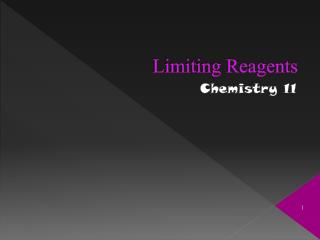DownloadDownload PresentationLimiting Reagents

# Limiting Reagents

Download Presentation## Limiting Reagents

- - - - - - - - - - - - - - - - - - - - - - - - - - - E N D - - - - - - - - - - - - - - - - - - - - - - - - - - -
##### Presentation Transcript

1. Limiting Reagents Chemistry 11

2. Why Do We Care? • Real reactions, in the lab, are rarely carried out in exact stiochiometric amounts • so... Chemists usually don’t mix reactants together in the exact amounts that chemical equations tell us to • Why not? • Synthetic chemistry is a lot like cooking

3. So What Is A Limiting Reagent? • Limiting Reagent- the reactant that is used up first is the limiting reagent • Excess Reagent- this reactant is present in greater quantities than necessary • By figuring out which reagent is limiting and which is excess we can determine how much product we SHOULD get

4. “I Don’t Follow....” • Okay, think of it like this: • To make one car you need 1 body and 4 tires:

5. Now, What If... • We have 2 car bodies and 4 tires? • We can still only make one car! • The second car body is an excess, the tires are limiting how many cars we can make!

6. Or, What If... • We have 1 car body and 8 tires? • We can still only make one car because we are now limited by the number of car bodies and have an excess of tires!

7. Lets Apply This To Chemistry • Think of the two reagents in terms of car bodies and tires • The number of moles tells us how much of each we have to start • Most of the time you are given the amounts of reagents in terms of grams, you need to CONVERT TO MOLES!! HINT GRAM

8. Okay, Lets Try It • Urea is prepared from the reaction of ammonia with carbon dioxide as follows: 2NH3(g) +CO2(g) (NH2)2CO(aq) + H2O(l) • If 637.2 g of NH3 are treated with 1142 g of CO2 which of the two reactants is the limiting reagent?

9. Step 1- Convert One Reactant to Moles 2NH3(g)+CO2(g) (NH2)2CO(aq) +H2O(l) • Convert to moles. 637.2 g 1142 g HAVE HAVE

10. Step 2- Calculate How Many Grams of Second Reactant Required 2NH3(g)+CO2(g) (NH2)2CO(aq) + H2O(l) 637.2 g 1142 g • Pick one (lets arbitrarily pick NH3) • Calculate the mols of CO2 necessary to react with the moles of NH3 we have • Mols NH3mols CO2 NEED =18.7 mols CO2

11. Step 3- Compare Need: 18.7 mols Have : 25.95 mols

12. Conclusion • So, since we have more CO2 than we actually need to do the reaction, CO2 is in excess and, hence, NH3 must be the limiting reagent.

13. General Steps: • 1. Convert both reactants to moles. • 2. See how many moles of the other reactant your need. • Mole bridge • 3. If you have more of a reactant than you need, it is in excess. If you have less than you need, it is limiting. • Ie. Look at which reactant limits the number of moles of product that you can make.